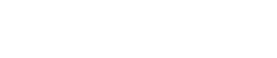# How to filter by column value

Here’s the tutorial on how to filter by column value. I show several ways to filter columns in Pandas.

Pandas offers many different ways to filter your data. I show how to filter a column using different methods in Pandas. Choose the filtering method that is most convenient for you.

## How to filter by column value

You can use the isin function to filter a column by the values in the column.

```import pandas as pd

my_values = ['5', '8']

my_df = pd.DataFrame({'Column1': ['2','7','6'],
'Column2': ['4','5','8'],
'Column3': ['6','4','3']})

print(my_df['Column2'].isin(my_values))

```You can find the documentation for the isin method at this link.

## How to filter row by column value

To find a row based on a column value you need to nest your data frame like below.

```import pandas as pd

my_df = pd.DataFrame({'Column1': ['2','7','6'],
'Column2': ['4','5','8'],
'Column3': ['6','4','3']})

print(my_df[my_df['Column2']=='5'])

```## How to filter column value by index

To find value based on column and index use python code like this.

```import pandas as pd

my_df = pd.DataFrame({'Column1': ['2','7','6'],
'Column2': ['4','5','8'],
'Column3': ['6','4','3']})

print(my_df['Column2'])

```## How to filter using loc

To filter a Pandas DataFrame by a column value, you can use the loc function to select rows based on a condition. Here is an example:

Assuming you have a DataFrame named df, and you want to filter it based on a column named column_name and a specific value value_to_filter.

```import pandas as pd

# create a sample DataFrame
data = {'column_name': [1, 2, 3, 4, 5],
'other_column': ['a', 'b', 'c', 'd', 'e']}
df = pd.DataFrame(data)

# filter the DataFrame by column value
filtered_df = df.loc[df['column_name'] == value_to_filter]```

In the above example, the loc function is used to select only the rows where the column_name is equal to value_to_filter. The resulting DataFrame, filtered_df, will contain only those rows.